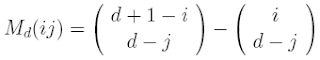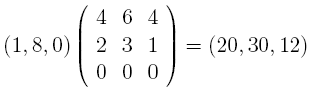The special \$M\$ matrices map the h-vector to the face vector as follows. First turn an h-vector into a g-vector by taking differences of entries. For example, \$(1,3,1)\$ will become \$(1,2)\$, because we imagine a dummy zero to the left of the h-vector. The vectors are shortened, because it is unnecessary to include the information that there is one cell for the polytope in the highest dimension. In dimension \$d\$, the \$M\$ matrix is defined bywhich leads to the odd convention of writing, for \$d = 3\$,The pentagon formula is given bywhich shows that a pentagon has \$5\$ vertices and \$5\$ edges. Our favourite associahedron in dimension \$3\$ is described byThese \$M\$ matrices work for any polytopes. Another example is the cyclohedron face vector, given byNote that the usual description of the \$M_d\$ matrices does not define them as square matrices, but square matrices work fine.